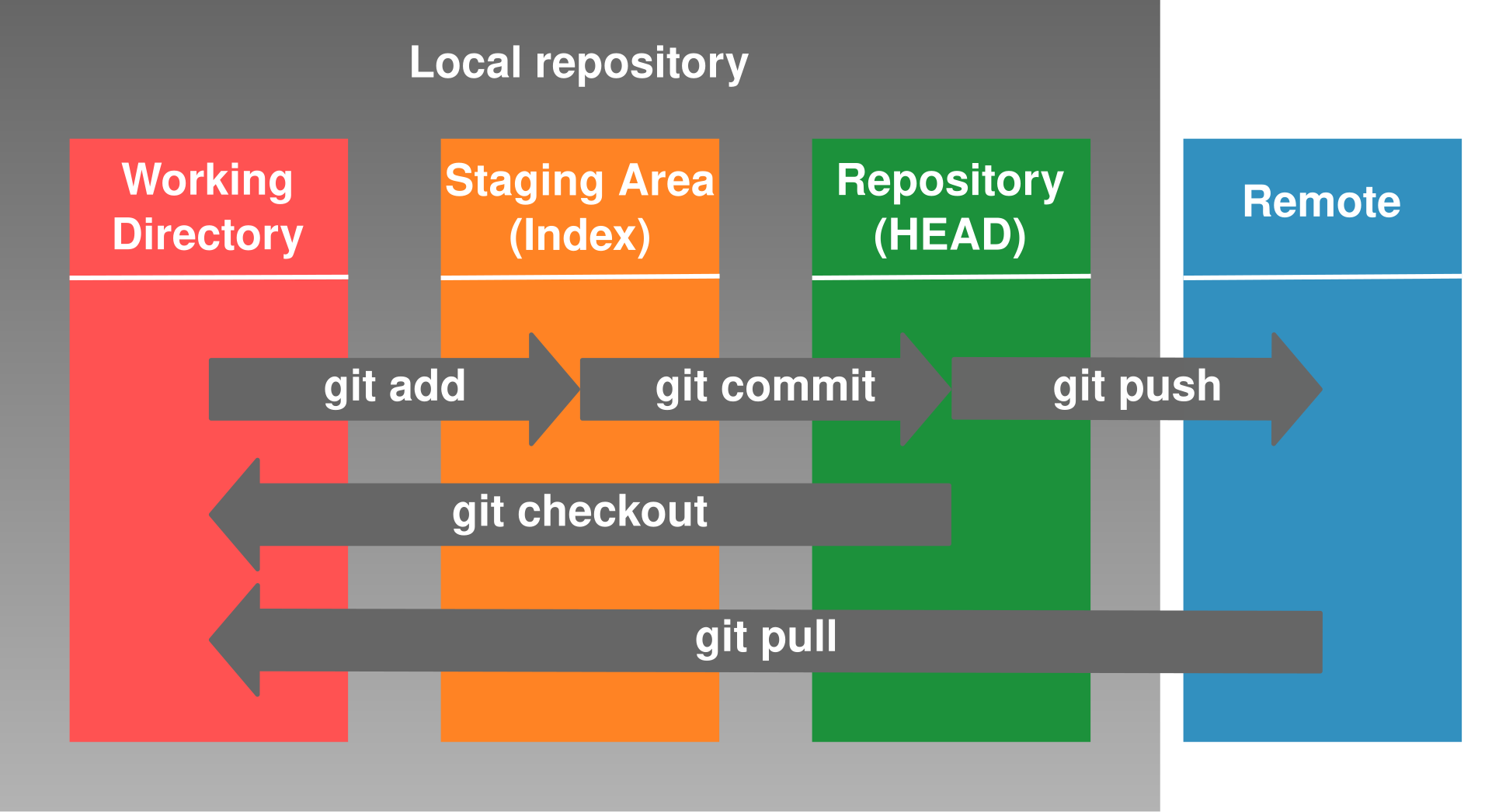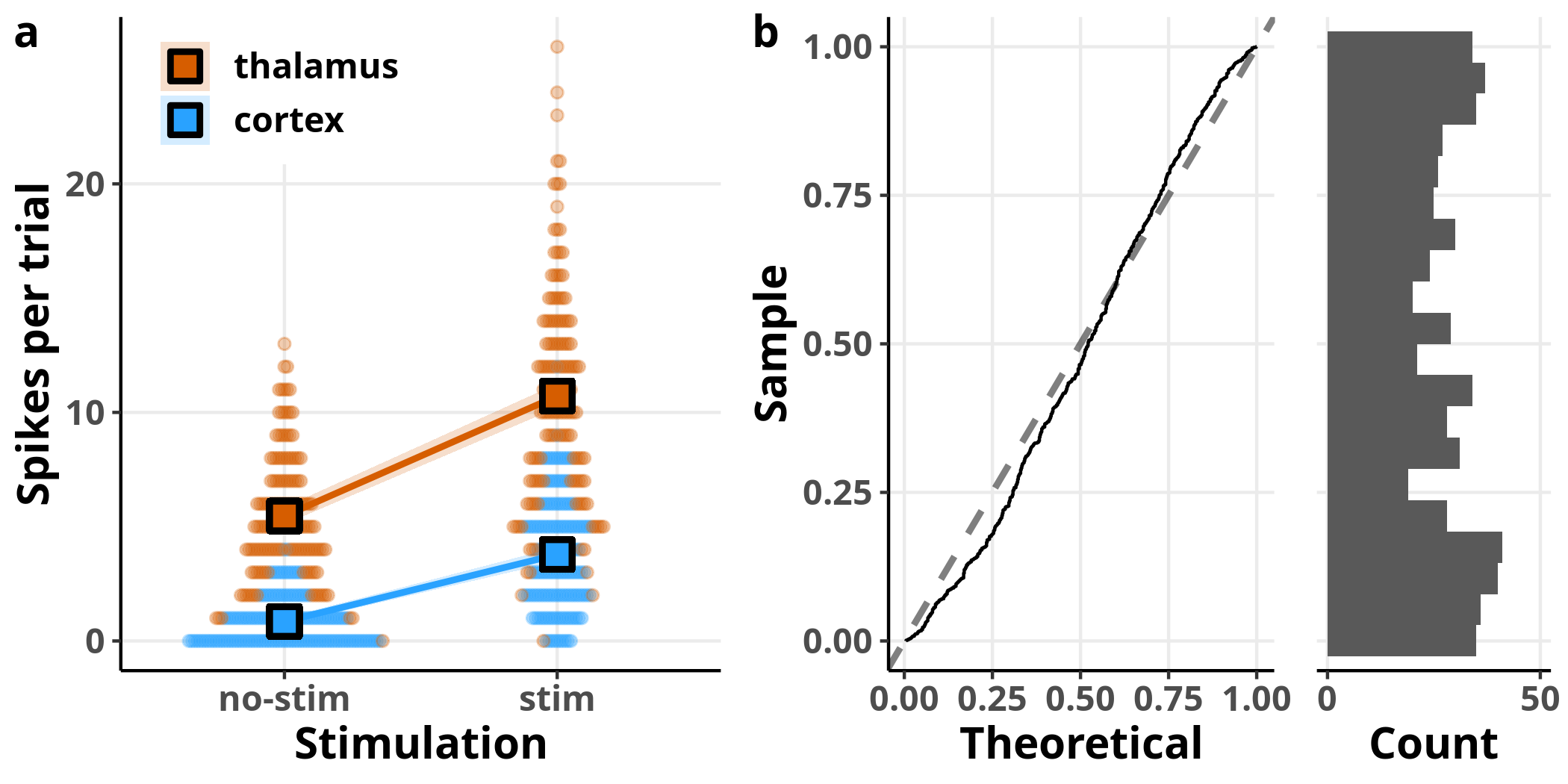Recent PostsProbability Theory

A simple set of unifying principles for statistical inferenceGit for scientists

A tool for source control, collaborative editing, and archiving

Image annotation with ScanImage in MATLAB

Longitudinal tracking of image featuresLinear models, Part II: Examples with R

Linear model examples with R

Linear models, Part I: Theory

A brief introduction into the theory behind linear models, including linear mixed-effects models and generalized linear (mixed) models